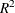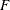# The SYSLIN Procedure

### Printed Output

Subsections:

The printed output produced by the SYSLIN procedure is as follows:

1. If the SIMPLE option is used, a table of descriptive statistics is printed that shows the sum, mean, sum of squares, variance, and standard deviation for all the variables used in the models.

2. If the FIRST option is specified and an instrumental variables method is used, first-stage regression results are printed. The results show the regression of each endogenous variable on the variables in the INSTRUMENTS list.

3. The results of the second-stage regression are printed for each model. (See the following section Printed Output for Each Model for details.)

4. If a systems method like 3SLS, SUR, or FIML is used, the cross-equation error covariance matrix is printed. This matrix is shown four ways: the covariance matrix itself, the correlation matrix form, the inverse of the correlation matrix, and the inverse of the covariance matrix.

5. If a systems method like 3SLS, SUR, or FIML is used, the system weighted mean squared error and system weightedstatistics are printed. The system weighted MSE andmeasure the fit of the joint model obtained by stacking all the models together and performing a single regression with the stacked observations weighted by the inverse of the model error variances.

6. If a systems method like 3SLS, SUR, or FIML is used, the final results are printed for each model.

7. If the REDUCED option is used, the reduced form coefficients are printed. These consist of the structural coefficient matrix for the endogenous variables, the structural coefficient matrix for the exogenous variables, the inverse of the endogenous coefficient matrix, and the reduced form coefficient matrix. The reduced form coefficient matrix is the product of the inverse of the endogenous coefficient matrix and the exogenous structural coefficient matrix.

#### Printed Output for Each Model

The results printed for each model include the analysis-of-variance table, the "Parameter Estimates" table, and optional items requested by TEST statements or by options in the MODEL statement.

The printed output produced for each model is described in the following.

The analysis-of-variance table includes the following:

• the model degrees of freedom, sum of squares, and mean square

• the error degrees of freedom, sum of squares, and mean square. The error mean square is computed by dividing the error sum of squares by the error degrees of freedom and is not affected by the VARDEF= option.

• the corrected total degrees of freedom and total sum of squares. Note that for instrumental variables methods, the model and error sums of squares do not add to the total sum of squares.

• theratio, labeled "F Value," and its significance, labeled "PROB>F," for the test of the hypothesis that all the nonintercept parameters are 0

• the root mean squared error. This is the square root of the error mean square.

• the dependent variable mean

• the coefficient of variation (CV) of the dependent variable

• thestatistic. Thisis computed consistently with the calculation of thestatistic. It is valid for hypothesis tests but might not be a good measure of fit for models estimated by instrumental variables methods.

• thestatistic adjusted for model degrees of freedom, labeled "Adj R-SQ"

The "Parameter Estimates" table includes the following:

• estimates of parameters for regressors in the model and the Lagrangian parameter for each restriction specified

• a degrees of freedom column labeled DF. Estimated model parameters have 1 degree of freedom. Restrictions have a DF of –1. Regressors or restrictions dropped from the model due to collinearity have a DF of 0.

• the standard errors of the parameter estimates

• the t statistics, which are the parameter estimates divided by the standard errors

• the significance of the t tests for the hypothesis that the true parameter is 0, labeled "Pr > |t|." As previously noted, the significance tests are strictly valid in finite samples only for OLS estimates but are asymptotically valid for the other methods.

• the standardized regression coefficients, if the STB option is specified. This is the parameter estimate multiplied by the ratio of the standard deviation of the regressor to the standard deviation of the dependent variable.

• the labels of the regressor variables or restriction labels

In addition to the analysis-of-variance table and the "Parameter Estimates" table, the results printed for each model can include the following:

• If TEST statements are specified, the test results are printed.

• If the DW option is specified, the Durbin-Watson statistic and first-order autocorrelation coefficient are printed.

• If the OVERID option is specified, the results of Basmann’s test for overidentifying restrictions are printed.

• If the PLOT option is used, plots of residual against each regressor are printed.

• If the COVB or CORRB options are specified, the results for each model also include the covariance or correlation matrix of the parameter estimates. For systems methods like 3SLS and FIML, the COVB and CORB output is printed for the whole system after the output for the last model, instead of separately for each model.

The third-stage output for 3SLS, SUR, IT3SLS, ITSUR, and FIML does not include the analysis-of-variance table. When a systems method is used, the second-stage output does not include the optional output, except for the COVB and CORRB matrices.